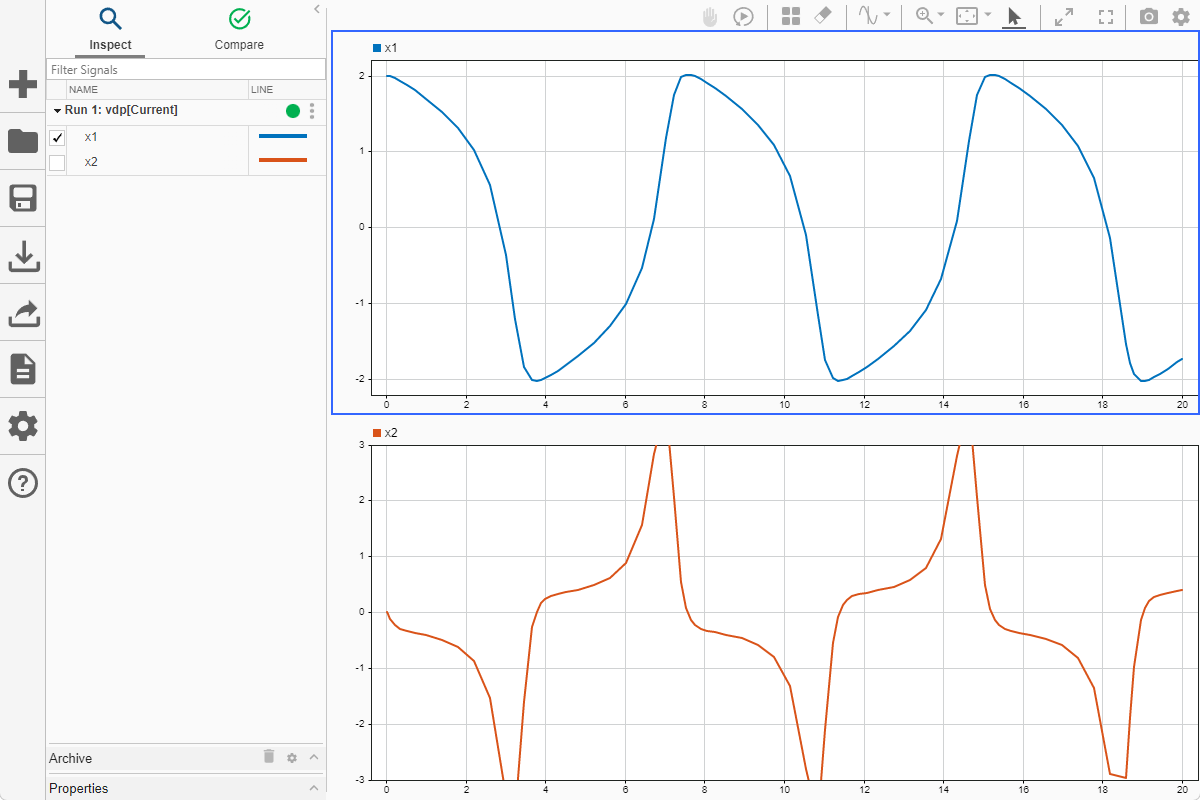Load a view file to visualize data in the Simulation Data Inspector

Since R2019b

## Syntax

``Simulink.sdi.loadView(filename)``

## Description

example

````Simulink.sdi.loadView(filename)` applies the visualization information in the view file, `filename`, to the data in the Simulation Data Inspector.A view contains information about the layout and plots in the graphical viewing area as well as properties of plotted signals. When you load a view, the Simulation Data Inspector uses the properties and preferences in the file to display data currently in the Simulation Data Inspector. Signals in the Simulation Data Inspector that align with the signals in the view are plotted in the graphical viewing area. Use a view to apply a consistent set of visualization settings to multiple sets of similar data.The view file includes the following information: Subplot layout and visualization typeSettings for each visualization type in the layoutSignal selection modeReplay controls visibilityMetadata displayed in the work areaSettings for signal grouping in the work areaPlotted signals and the line style and color for each plotted signal A view file does not contain data. To load a session file that contains data and visualization information, use the `Simulink.sdi.load` function.```

## Examples

collapse all

This example visualizes the output from the `ex_vdp` model, saves the configuration as a view, and then uses the view to visualize the output from another simulation of the same model.

To generate data to plot in the Simulation Data Inspector, simulate the model.

```open_system('ex_vdp') set_param('ex_vdp/Mu','Gain','1') sim('ex_vdp');```

Programmatically Configure and Save a View

The `ex_vdp` model is configured to log two signals, `x1` and `x2`. Plot each signal on a subplot in a `2`-by-`1` layout.

`Simulink.sdi.setSubPlotLayout(2,1)`

To plot one signal on each subplot, first access the `Simulink.sdi.Run` object for the simulation.

```runIDs = Simulink.sdi.getAllRunIDs; ex_vdpRunID = runIDs(end); ex_vdpRun = Simulink.sdi.getRun(ex_vdpRunID);```

Then, you can access the data for each signal in a `Simulink.sdi.Signal` object and use the `plotOnSubPlot` function to specify where to plot each signal.

```x1 = getSignalByIndex(ex_vdpRun,1); x2 = getSignalByIndex(ex_vdpRun,2); plotOnSubPlot(x1,1,1,true) plotOnSubPlot(x2,2,1,true)```

To view the result in the Simulation Data Inspector, enter `Simulink.sdi.view` in the command window.Then, save the view.

`Simulink.sdi.saveView('ex_vdpView.mldatx')`

Use the View

To mimic a situation where the Simulation Data Inspector is configured differently and contains data from another simulation, this example clears data from the Simulation Data Inspector, loads a view that represents the default visualization configuration, and runs another simulation of the `ex_vdp` model.

```Simulink.sdi.clear Simulink.sdi.loadView('default.mldatx'); set_param('ex_vdp/Mu','Gain','2') sim('ex_vdp');```

Use the `Simulink.sdi.loadView` function to apply the saved view from the previous simulation.

`Simulink.sdi.loadView('ex_vdpView.mldatx')`

You can open the Simulation Data Inspector to view the results using the `Simulink.sdi.view` function. The saved view includes information regarding the axes limits. When you use a view to apply visualization settings, you may need to adjust axes limits or zoom levels to fit data from a modified simulation.## Input Arguments

collapse all

Name of the view file, specified as a string or character vector. You can specify `filename` as only the file name when the file is on the MATLAB® path, or you can specify a full path to the file.

Example: `'myView.mldatx'`

Data Types: `char` | `string`

## Version History

Introduced in R2019b